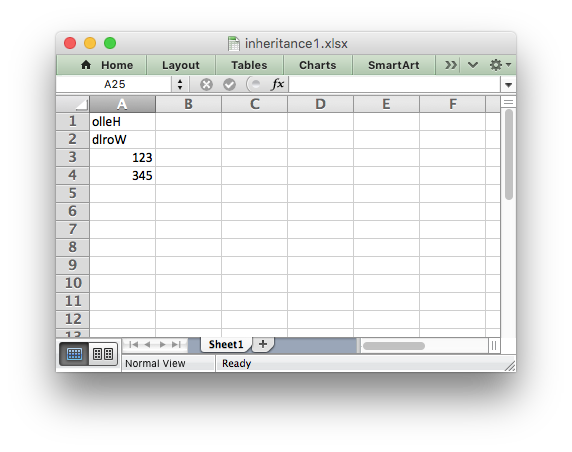# Example: Example of subclassing the Workbook and Worksheet classes

Example of how to subclass the Workbook and Worksheet objects.

We also override the default `worksheet.write()` method to show how that is done.```##############################################################################
#
# Example of how to subclass the Workbook and Worksheet objects. We also
# override the default worksheet.write() method to show how that is done.
#
# Copyright 2013-2022, John McNamara, jmcnamara@cpan.org
#
from xlsxwriter.workbook import Workbook
from xlsxwriter.worksheet import Worksheet
from xlsxwriter.worksheet import convert_cell_args

class MyWorksheet(Worksheet):
"""
Subclass of the XlsxWriter Worksheet class to override the default
write() method.

"""

@convert_cell_args
def write(self, row, col, *args):

data = args

# Reverse strings to demonstrate the overridden method.
if isinstance(data, str):
data = data[::-1]
return self.write_string(row, col, data)
else:
# Call the parent version of write() as usual for other data.
return super(MyWorksheet, self).write(row, col, *args)

class MyWorkbook(Workbook):
"""
Subclass of the XlsxWriter Workbook class to override the default
Worksheet class with our custom class.

"""

# Overwrite add_worksheet() to create a MyWorksheet object.
worksheet = super(MyWorkbook, self).add_worksheet(name, MyWorksheet)

return worksheet

# Create a new MyWorkbook object.
workbook = MyWorkbook('inheritance1.xlsx')

# The code from now on will be the same as a normal "Workbook" program.